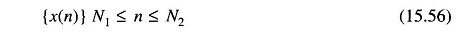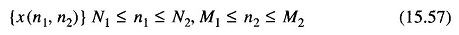## Properties of Linear Digital Filters:

The properties of linear digital filters defined as transformations of discrete functions are described in the following. Discrete Functions Equation are defined only for discrete values of their variables, that is, they are sequences of numbers. These numbers can be obtained as quantized samples of continuous functions (representing an analog signal or image) or they can be values of a discrete variable, such as the readings indicated by, or the output data of a digital counter. The sequences thus obtained are then processed to obtain other sequences.

The notation which is useful for one dimensional (1-D) sequences is the followingwhere N1 = – ∞ and N2 = ∞ for infinite sequences.

For two dimensional (2-D) sequences, the notation iswhere N1 = M1 = – ∞ and N2 = M2 = ∞ for double infinite sequences.

We can observe that with the terms, the sequence number and numerical transformation of sequences, the quantization aspect is conceptually included due to the implicit finite precision needed for any numerical representation. However, in the development of the theory of discrete linear systems we consi­der the more general case of sequences of numbers with infinite precision. Thus we use functions whose variable is defined on a discrete set of values, but whose amplitude can assume values in a continuous way within a specified range.

In many applications, sequences of the type (1-D) and (2-D) are obtained by taking samples of continuous or analog signals and images. Therefore, we consider, in greater precision the way an analog signal is related to its sample, leading to the sampling theorem.

Scroll to Top